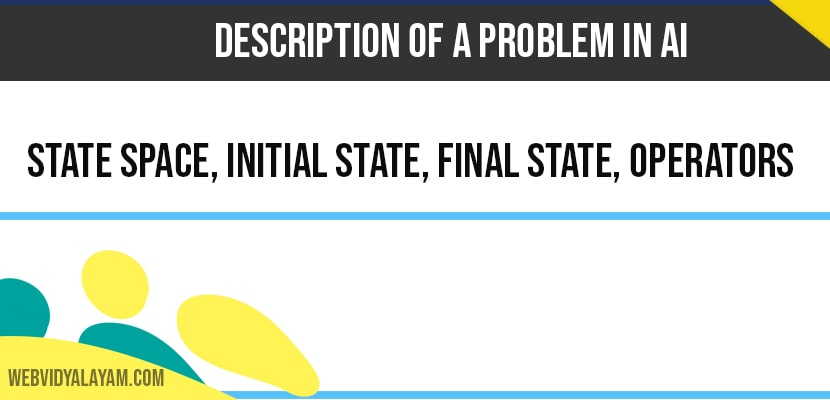# Description Of a Problem in AI (Artificial Intelligence)

Description of Problems in AI consists of 4 states as below

1) State Space

2) Initial State

3) Final State

4) Operators

## State Space

State space: The state space is defined as the set of all possible configurations of a relevant object.

## Initial State

Initial state: Initial state specifies one or more states within that space as a possible situations from which the problem solving starts.

## Final State

Final state: Final state specify one or more states that could be acceptable as solutions to the given problem.

## Operators

Operators: Operators are the set of rules that describe how we are going to move from one state to other.

The above is the formal description of problem solving a state space search First we have to define state space i.e., all the possible configurations. We then identify one or more states within the space as possible situations from which we can start. This is the initial state from which one or more states from the problem space that are acceptable as solutions to the given problem is referred as final state operators have two portions one is right and the other is left.

As we know that operators are the set of rules which describes how we are moving from one state to other This information regarding the move must be true. For suppose in the above example of 3*3 matrix. In this case we have 8 tiles with information and one with empty space this empty space is a problem state. Some rules are specified to this problem state like move right or left or up or down.

Here if we apply any one among the rules lets say like to move right. It can be done and by this action new state space is generated. The second rule is to move left here in this case the second rule is not applicable. So when we specify the rules that describes actions it must be true for the action to take place needs to be looked at first and then the action can take place and generates new state space.

Start ‘S’
2 8 3
1 6 4
7 5
Destination ‘D’
1 2 3
8 4
7 6 5